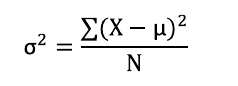Tags
#has-images
Question
What is the formula for the variance and the Standard Deviation of a given value in a sample?Which means : (sum of squares - (square of the sum/number of items))/(number of items - 1)

Tags
#has-images
Question
What is the formula for the variance and the Standard Deviation of a given value in a sample?
?

Tags
#has-images
Question
What is the formula for the variance and the Standard Deviation of a given value in a sample?Which means : (sum of squares - (square of the sum/number of items))/(number of items - 1)
If you want to change selection, open document below and click on "Move attachment"

#### pdf

owner: naxplast06 - (no access) - Deviant S. - The Practically Cheating Statistics Handbook (2010, CreateSpace Independent Publishing Platform).pdf, p35

#### Summary

status measured difficulty not learned 37% [default] 0

No repetitions